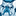# New to Qlik Sense

If you’re new to Qlik Sense, start with this Discussion Board and get up-to-speed quickly.

Announcements
cancel
Showing results for
Did you mean:Contributor II

## Create filter or button

Hi

im unable to reply in this post  https://community.qlik.com/t5/New-to-Qlik-Sense/count-in-range/m-p/1881572#M198610.

I have to create a filter or button like below

Mon-Fri    and  Sat

so if user selects  Mon-Fri it will display in KPIS or charts  those related data

if user select on sat  it will display only sat data.

any suggestions ?

Labels (3)

• ### Visualization

2 Solutions

Accepted SolutionsCreator II

Hello,

create a variable name it e.g. vKPIValue, set it's value to 0.

Create a KPI object and write the following:

``````=IF(\$(vKPIValue)=0,
Count({<DayName={'Mon', 'Tue', 'Wed', 'Thu', 'Fri'}, Time={">=\$(=Num(MakeTime(8,30,0)))<=\$(=Num(MakeTime(20,0,0)))"}>}Date),
Count({<DayName={'Sat'}, Time={">=\$(=Num(MakeTime(8,30,0)))<=\$(=Num(MakeTime(13,30,0)))"}>}Date)
)``````

If you don't need the time restraint, simply remove it from the set analysis.

Create two buttons and give it the vKPIValue as the variable you want to change.

Name it Mon-Fr and it's value 0 and the other Sat and it's value 1 for example.Creator II

Sure. The table that contains our date value needs another field DayName.

You can try in script:

``````if(Weekday(Date)='Sat', 'Sat',
if(Match(Weekday(Date),'Mon','Tue','Wed','Thu','Fri')>0, 'Mon-Fri')) as DayName``````

5 RepliesCreator II

Hello,

create a variable name it e.g. vKPIValue, set it's value to 0.

Create a KPI object and write the following:

``````=IF(\$(vKPIValue)=0,
Count({<DayName={'Mon', 'Tue', 'Wed', 'Thu', 'Fri'}, Time={">=\$(=Num(MakeTime(8,30,0)))<=\$(=Num(MakeTime(20,0,0)))"}>}Date),
Count({<DayName={'Sat'}, Time={">=\$(=Num(MakeTime(8,30,0)))<=\$(=Num(MakeTime(13,30,0)))"}>}Date)
)``````

If you don't need the time restraint, simply remove it from the set analysis.

Create two buttons and give it the vKPIValue as the variable you want to change.

Name it Mon-Fr and it's value 0 and the other Sat and it's value 1 for example.Contributor II
Author

Hi,

Instead of doing with set analysis. Can i do it in script create field values like

Mon-Fri

Sat

so that if user selects that data will display .Creator II

Sure. The table that contains our date value needs another field DayName.

You can try in script:

``````if(Weekday(Date)='Sat', 'Sat',
if(Match(Weekday(Date),'Mon','Tue','Wed','Thu','Fri')>0, 'Mon-Fri')) as DayName``````Contributor II

I asked a similar question yesterday here

=Pick(Match(FieldName,'Mon','Tue','Wed','Thur','Fri','Sat'), 'Mon-Fri', 'Mon-Fri', 'Mon-Fri', 'Mon-Fri', 'Mon-Fri', 'Sat')Contributor II
Author